Degree Of Saturation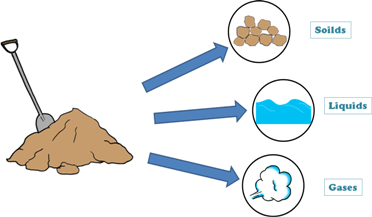A soil mass in general is composed of three kinds of matter,

1. Solids

2. Liquid

3. Gaseous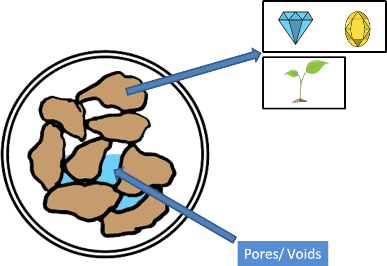Solids can be minerals or organic matters or both. These solids can be of different sizes and shapes and because of it they enclose empty spaces. These empty spaces are called pores or voids.

The proportions of solids, water and air in a soil mass influence its physical properties. And before founding any structure on the soil we should study the soil in detail including its physical properties.

To determine these physical properties of soil we need to learn few simple terms which are frequently used in soil engineering.

Lets discuss one of these soil properties: Degree of saturation.

Degree of saturation can be understood as amount of empty space present in the soil sample is filled with water. If this space, which is called pores or voids, is completely filled with water then soil is called fully saturated. If no water is present in this space then soil is called dry.

And if this empty space is partly filled then the soil sample is called partially saturated soil sample.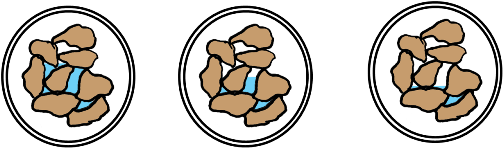All of the soil samples in the figure represent partially saturated soils. All these soils have different amount of water in them.

We can see there is clearly a need to define the term “Degree of Saturation” to differentiate between these states of soil and to know that how much partially saturated soil is saturated.

So let us define the term Degree of Saturation which is denoted by capital letter S.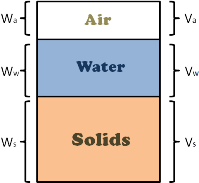We can imagine a soil mass with its constituents (i.e. solids, water and air) separated, though these different constituents present in the soil mass cannot be separated, but it will be helpful in understanding the soil behavior and deriving its properties.

We can think of constituents occupying separate spaces. Volume of solids in the soil mass is marked as Vs, volume of water is as Vw and volume of air as Va.

Such diagrammatic representation of separated different phases of soil mass is called phase diagram.

Let us consider the 3 phase diagram of a partially saturated soil.

Degree of saturation for this soil sample is defined as volume of water to the volume of voids this soil sample contains.

Degree of saturation sometimes represented as percentage and sometimes doesn’t.

In the equation form it can be written as this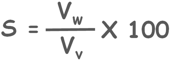Since Degree of Saturation indicates the portion of volume of voids which is filled with water, so when soil doesn’t have any water in it then value of Vw in equation becomes zero and hence Degree of Saturation comes as zero.

And similarly when all the pores are filled with water then Volume of voids is equal to volume of water present in the soil, so in the equation value of this ratio becomes 1 and Degree of Saturation comes hundred percent or simply 1 if not shown as percentage.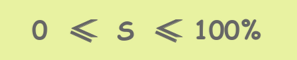Tags : saturation

Published on :2019-08-04